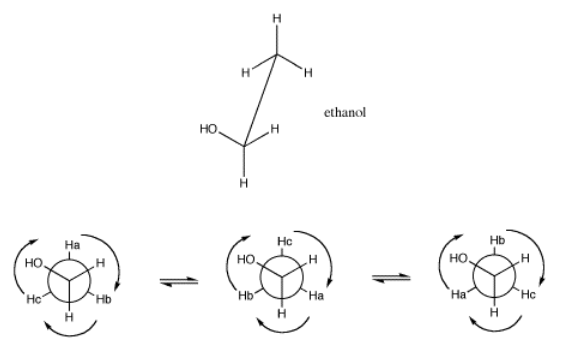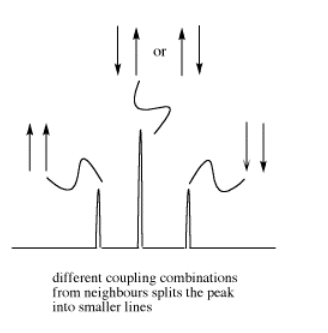Skip to main content

# 4.11: More Complicated Coupling

The n + 1 rule (number of lines in a multiplet = number of neighbouring H + 1) will work for the majority of problems you may encounter. Occasionally, you may see more complicated coupling. The spectrum of methyl acrylate is a good example. There are a couple of points to note in this spectrum, beginning with the number of peaks.Figure $$\PageIndex{1}$$: 1H NMR spectrum of methyl acrylate.

Source: Simulated spectrum.

• the vinyl portion of the spectrum (hydrogens adjacent to the C=C double bond) shows three peaks, not just two.
• although there are two protons on one vinyl carbon, each H is different because of cis/trans relationships: one H is cis to the carbonyl and the other is trans to it.
• these hydrogens are symmetry inequivalent.

In addition, there is a problem with coupling in the vinyl region.

• the proton adjacent to the carbonyl, at 6 ppm, has two neighbors and should give a triplet.
• instead, the peak from this proton shows four lines, not three.
• this peak is not a quartet; the middle two peaks are not three times as tall as the edge ones.
• the lines within this multiplet are symmetrically spaced, but not evenly; the middle space is smaller than the spaces on the edges.

This pattern is called a "doublet of doublets". The two symmetry-inequivalent neighbors on the other end of the double bond each act as if the other one isn't there. They couple to the proton next to the carbonyl independently, each one splitting the peak for this proton into a separate doublet.

There are a few cases in which this independent coupling will occur rather than the (n+1) type coupling we saw first. Generally, independent coupling occurs when protons are not freely rotating. That can happen if one of the protons is attached to a double-bonded carbon, because we can't rotate around a double bond. It may also happen with protons that are directly attached to the carbons of a ring.

To see why this happens, you need to know more about coupling.

• in coupling, magnetic information is shared between two protons. How fully this information is shared depends on the angle between the hydrogens as you look down the connecting C-C bond.
• for (n+1) coupling, the rotational angle about the C-C bond connecting the two protons (or 'dihedral angle' between the coupled hydrogens) must be unrestricted. The bond must be able to rotate freely.
• if the bond can rotate freely, any proton on the neighbouring carbon can assume any dihedral angle with the proton being observed. That means coupling information from one neighbouring proton is indistinguishable from another, and so all the neighbouring protons affect the observed proton equally.Figure $$\PageIndex{2}$$: A sawhorse projection (top) and Newman projection of ethanol (bottom), showing the angular relationship between hydrogens on neighbouring carbons. The angle between two groups on neighbouring carbons is called the dihedral angle. Because of free rotation about the C-C bond, all three hydrogens on the methyl have an equivalent spatial relationship to the hydrogens on the CH2 group.

Sometimes coupling information is depicted as an arrow. This arrow stands for the coupling constant between two protons. The coupling constant is related to the spin of a hydrogen atom. The spin (related to magnetic moment) can be aligned with the external magnetic field (we will show it pointing up) or else against it; no other possibilities are allowed.If there are two neighbouring hydrogens, both spins could be aligned with the external field, both could be aligned against it, or one could be aligned each way. That means there are three different magnetic combinations that will each have a different effect on the observed proton: increased magnetic field, decreased magnetic field, and no net effect (canceling out).These three combinations result in the observed proton absorbing at three different frequencies, because the frequency it absorbs is sensitive to the magnetic field it experiences. Note that there are two ways to arrive at the middle possibility, with one neighbour spin up and the other spin down. Statistically this possibility is twice as likely as either both spins up or both spins down. It is thus twice as likely that the observed proton experiences that effect, and so the middle line in a triplet is twice as high as the other two lines.Figure $$\PageIndex{3}$$: Effects of neighbouring protons on an observed peak. This case assumes all the neighbouring protons have an equivalent effect (they have the same coupling constant with the observed proton).

However, the size of that arrow, the coupling constant, is only the same for two neighbouring hydrogens if they have the same spatial relationship with the observed hydrogen. That isn't always true.

• if the dihedral angle is limited, complex coupling occurs.
• complex coupling occurs because one neighbouring hydrogen can only adopt a limited range of dihedral angles. Another neighbouring proton can adopt a limited range of dihedral angles as well, but these two ranges do not overlap.

As a result, the two coupling constants are different. We can depict that situation using arrows of different lengths for the two neighbouring proton spins. Each spin can be either up or down, but now two opposing spins do not cancel out. The result is four spin combinations of equal probability, not just three.The doublet of doublets is four lines of about equal heights. The distance between the two pairs of lines on each edgeFigure $$\PageIndex{4}$$: Effects of neighbouring protons on an observed peak. This case assumes neighbouring protons have inequivalent effects (they have differing coupling constants with the observed proton).

The dihedral angle is limited in only a few specific cases:

• there is a double bond. Double bonds cannot rotate because that would require breaking the pi bond. In a pi bond, p orbitals on the two carbons must remain coplanar in order to overlap and form a bond.
• there is a ring. In a ring, there can't be complete rotation about a bond because the ring would twist into a pretzel.
• in some cases, there can be such large groups of atoms on either end of a bond that it is difficult to rotate the bond without having these groups crash into each other.

Exercise $$\PageIndex{1}$$

Sketch spectra for the following compounds.

a) allyl alcohol or 2-propene-1-ol, HOCH2CH=CH2

b) styrene or vinylbenzene, C6H5CH=CH2

c) trans-1-chloropropene, CH3CH=CHCl

Exercise $$\PageIndex{2}$$

A compound was shown via high-resolution mass spectrometry to have the probable formula C6H10O2.

a) What is the degree of unsaturation in this compoud?

b) IR spectroscopy gave the following data: 3105 (w), 2950 (m), 1517 (m), 1235 (s), 1056 (s), 715 (m) cm-1. Provide a data table with possible assignments for these peaks.

c) 1H NMR spectroscopy provided the following spectrum. Provide a data table with partial structures for each peak.d) Suggest a likely structure for this compound

Exercise $$\PageIndex{3}$$

A compound was shown via high-resolution mass spectrometry to have the probable formula C5H8O2.

1. What is the degree of unsaturation in this compound?
2. IR spectroscopy gave the following data: 2950 (m), 2825 (m), 2716 (m), 1724 (s), 1505 (m), 1056 (w) cm-1. Provide a data table with possible assignments for these peaks.
3. 1H NMR spectroscopy provided the following spectrum. Provide a data table with partial structures for each peak.4. Suggest a likely structure for this compound.

Exercise $$\PageIndex{4}$$

A sample of a natural product was isolated from plant material and subjected to analysis.

1. IR spectroscopy gave the following data: 3097 (m), 2975 (m), 1600 (m), 1495 (m), 1235 (s), 1056 (s), 747 (m), 705 (m) cm-1. Provide a data table with possible assignments for these peaks.
2. The compound was shown via high-resolution mass spectrometry to have the probable formula C10H12O. What is the degree of unsaturation in this compound?
3. 1H NMR spectroscopy provided the following spectrum. Provide a data table with partial structures for each peak.4. Suggest a likely structure for this compound.